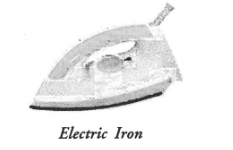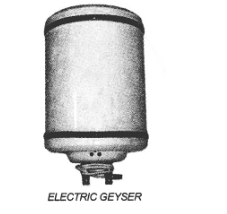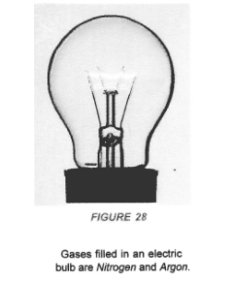# What is Joule’s heating effect ?

Question:

What is Joule’s heating effect ? How can it be demonstrated experimentally ? List its four applications in daily life.

(CBSE 2012)

Solution:

Joule’s law can be stated as : The amount of heat produced in a conductor is

(i) directly proportional to the square of the electric current flowing through it.

This is                  H ∝ I2                                                           –

(ii) directly proportional to the resistance of the conductor or resistor.

That is,                H ∝ R                                          ‘

(iii) directly proportional to the time for which the electric current flows through the conductor or resistor.

That is,               H ∝ t

Combining (i), (ii) and (iii), we get H ∝ I2 Rt.

or         H = KI2Rt, where K is constant of proportionality

If          K = 1, then H = I2Rt joule

This is known as Joule’s law of heating.

Four Applications:

1. When electric appliances like electric heater, electric iron and water heater etc. are connected to the main supply of electricity, these appliances become hot but the connecting wires remain cold.
We know, heat produced is directly proportional to the resistance of the material through which current flows. The element of electric heater is made of nichrome. Since, resistance of nichrome is high, so a large amount of heat is produced in the element of the electric heater. Thus, filament of electric heater becomes red hot. However, heat produced in connecting wires made of copper or aluminium is very small and hence they are not heated up.2. Filament of an electric bulb is made of a thin wire of tungsten. The melting point of filament is high i.e., about $3380^{\circ} \mathrm{C}$. The filament of the bulb is enclosed in a glass envelope fixed over an insulated support as shown in figure 28 . The glass envelope of electric bulb is filled with inactive gases like nitrogen and argon to increase the life of the tungsten filament.

Since resistance of thin filament is very high, so a large heat is produced as the electric current flows through the filament. Due to this large amount of heat produced, filament of the bulb becomes white hot. Hence, the filament of the bulb emits light and heat.3. Electric fuse is a safety device connected in series with the electric circuit. Electric fuse is a wire made of a material whose melting point is very low. Examples of the materials of making fuse wire are copper or tin-lead alloy. When large current flows through a circuit and hence through a fuse wire, a large amount of heat is produced. Due to this large amount of heat, the fuse wire melts and the circuit is broken so that current stops flowing in the circuit. This saves the electric circuit from burning due to the passage of large current through it.